## 31 Mar 2016

### Lawvere & Schanuel (Preview) Conceptual Mathematics: A First Introduction to Categories, “Galileo and Multiplication of Objects”, summary

by Corry Shores
[Search Blog Here. Index-tags are found on the bottom of the left column.]

[The following is summary. All boldface and bracketed commentary is mine.]

Entry Directory for

F. William Lawvere & Stephen H. Schanuel

Conceptual Mathematics: A First Introduction to Categories

Preview

Session 1: Galileo and Multiplication of Objects

Brief Summary:

In this book we explore the notion of category in terms of objects, maps, and map composition. We are here first given a glimpse with the example of maps that “multiply” domains, as in the case of motion being decomposed into domains of temporal and spatial locations, the latter of which being decomposed into multiple spatial dimensions.

Summary

1. Introduction

In this book, the authors will “explore the consequences of a new and fundamental insight about the nature of mathematics which has led to better methods for understanding and using mathematical concepts” (3). They continue:

The basic notion which underlies all the others is that of a category, a ‘mathematical universe’. There are many categories, each appropriate to a particular subject matter, and there are ways to pass from one category to another.
(3)

So they will begin with an informal introduction that will provide examples of objects, maps, and composition of maps.

Although these notions were used informally one way or another throughout the history of mathematics, it was not until 1945 that Eilenberg and MacLane gave explicit definitions to these basic concepts (3)

2. Galileo and the Flight of a Bird

Galileo wanted to understand the motion of objects. Motion is more than just the track of locations an object makes, like the parabolic arc of a thrown rock. “The motion involves, for each instant, the position of the rock at that instant; to record it requires a motion picture rather than a time exposure. We say the motion is a ‘map’ (or ‘function’) from time to space” (3).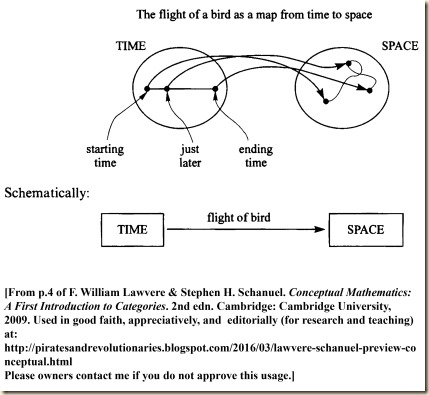As the legend goes, Galileo dropped both a heavy and a light weight simultaneously from the leaning tower of Pisa. Spectators were surprised to see that they both landed at the same time. But this motion is not so interesting, because the object moves in a straight line. The reason the authors say Galileo chose it has to do with the simple equation:

SPACE = PLANE × LINE

The authors will now explain.

We will consider two new maps. We first are to imagine that the sun is directly overhead. So for each point in space [intervening between us and the sun] we will have a shadow point on the horizontal plane [the ground below us].This shadow map from space to the plane is our first map. Now for the second map, we need to think of a pole sticking upright in the ground, because we need now a vertical line. [So the horizontal plane gives us the coordinates of the point as they spread out horizontally, but without indication of how high or how low the point is. The vertical line will now indicate how high or low, but not where the point is on a horizontal plane.]

The second map we need is best imagined by thinking of a vertical line, perhaps a pole stuck into the ground. For each point in space there is a corresponding point on the line, the one at the same level as our point in space. Let’s call this map ‘level’:
(3)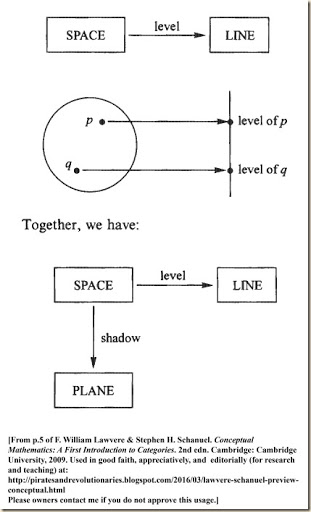So now we have two maps, the “shadow” map and the “level” map.  Together these two maps “seem to reduce each problem about space to two simpler problems, one for the plane and one for the line” (5). So we can determine a bird’s location in our space by knowing where its shadow is and what level it is at. [The next example is more complicated. For this we will trace the entire path of a bird flying through the space. We need two cameras for this, but how it all works out I am not sure. We need one camera-person who keeps aimed at the flying bird, but this camera-person moves up and down, staying level with the bird. Simultaneously we need another camera-person filming the first camera-person. I do not know how the footage will be combined to trace the flight, however. How does the level-changing camera-person capture the horizontal position? Would she not need to be above the bird to know the horizontal position, since the plane is two dimensional? Let me quote:]

Suppose you have a motion picture of the bird’s shadow as it flies, and a motion picture of its level – perhaps there was a bird-watcher climbing on our line, keeping always level with the bird, and you filmed the watcher. From these two motion pictures you can reconstruct the entire flight of the bird! So not only is a position in space reduced to a position in the plane and one on the line, but also a motion in space is reduced to a motion in the plane and one on the line.
(5)

[Recall this chart from before:This very generally gave us a relation between the time and space of the bird’s flight. We then decomposed the spatial component into two parts, the horizontal plane (which has two dimensions) and the vertical part (with one dimension). The authors will show how the original double mapping of time and space, when combined with the double mapping of space to plane and line, makes a triple mapping that then compresses to a double map of time to plane and line. ]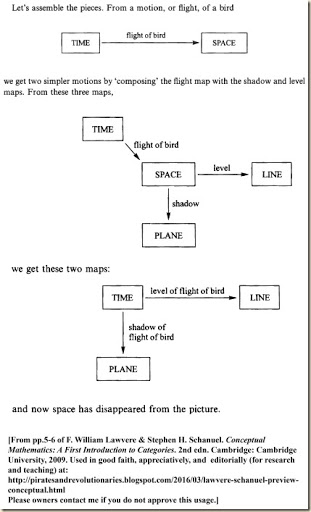Using this mapping, Galileo was able “to reduce the study of motion to the special cases of horizontal and vertical motion” (6).

The authors then ask if it is reasonable to use the equation SPACE = PLANE × LINE to express the relation of the two maps in this diagram.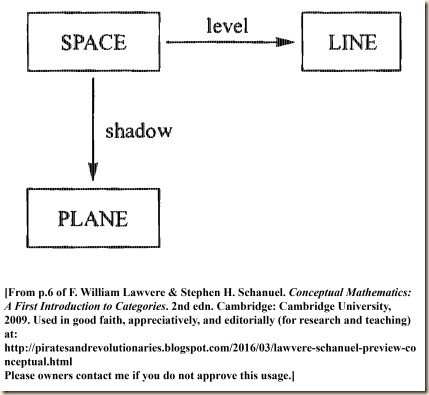They then wonder, “What do these maps have to do with multiplication?” To answer this, they will examine more examples.

3. Other Examples of Multiplication of Objects

They say that multiplication “often appears in the guise of independent choices” (7). They offer the example of option lists on restaurant menus. There may be a number of courses, and we choose an option from one list for the first course, and then another option from another list for the second course. A meal is comprised of one item from each list. The options for the first course are: soup, pasta, or salad. And for the second course: steak, veal, chicken, or fish.

We could for example make a meal as “soup, then chicken.” But one meal we cannot make is “veal, then steak” [since they are both on the same list, and nothing was chosen from the other list] (7). The authors then provide this diagram for possible meals, and they also show the mapping is comparable to the Galileo chart: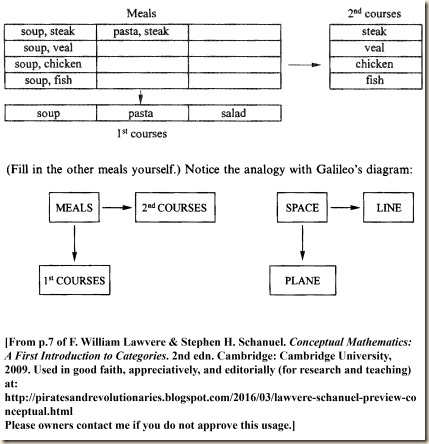So here we have a scheme with three “objects” and two “maps” or “processes”. This is a multiplication of objects. We find it operating in many different situations. In fact, a cylinder can be understood as the multiplication of a disc with its various levels. This is not just finding volume by multiplying these dimensions. Rather, the cylinder is composed by a process of multiplying the components.

Take for example a segment and a disk from geometry. We can multiply these too, and the result is a cylinder. I am not referring to the fact that the volume of the cylinder is obtained by multiplying the area of the disk by the length of the segment. The cylinder itself is the product, segment times disk, because again there are two processes or projections that take us from the cylinder to the segment and to the disk, in complete analogy with the previous examples. | Every point in the cylinder has a corresponding ‘level’ point on the segment and a corresponding ‘shadow’ point in the disk, and if you know the shadow and level points, you can find the point in the cylinder to which they correspond. As before, the motion of a fly trapped in the cylinder is determined by the motion of its level point in the segment and the motion of its shadow point in the disk.
(7-8)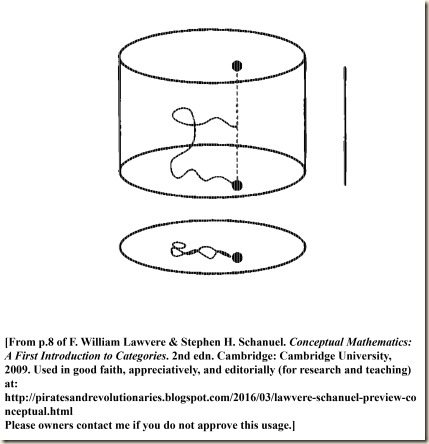The authors then suggest that we can understand multiplication in terms of the conjunctive operator in logic, “and” (8). We will take a conjunction of the form, A and B, for example, “John is sick and Mary is sick” (8). From this we can deduce A and as well we can deduce B.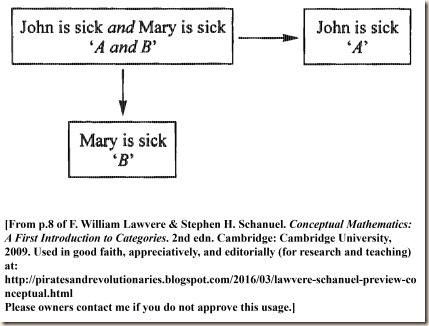[The next point I do not follow so well. It seems they are are saying that we already inferred the original “John is sick and Mary is sick” from yet another sentence, but I am not sure. Let me quote so you can interpret it:]

But more than that: to deduce the single sentence ‘John is sick and Mary is sick’ from some other sentence C is the same as deducing each of the two sentences from C. In other words, the two deductions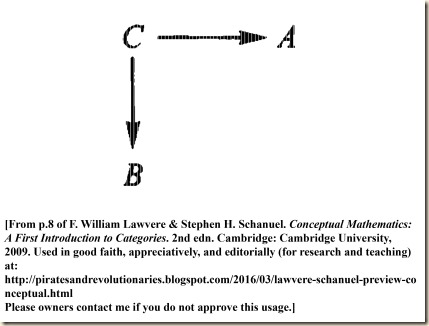amount to one deduction C → (A and B). Compare this diagram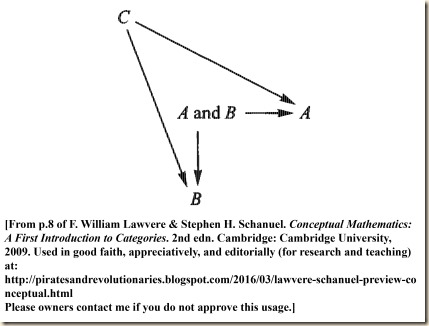with the Diagram of Galileo’s idea.
(8)

The authors then give one last diagram, this time for numerical multiplication.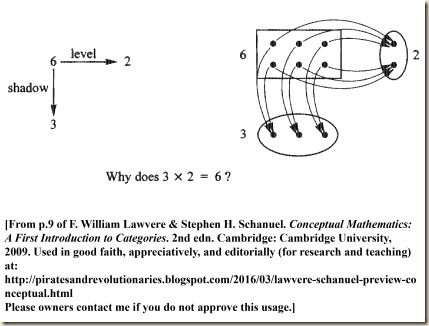The authors conclude by writing: “I hope these pictures seem suggestive to you. Our goal is to learn to use them as precise instruments of understanding and reasoning, not merely as intuitive guides” (9).

F. William Lawvere & Stephen H. Schanuel. Conceptual Mathematics: A First Introduction to Categories. 2nd edn. Cambridge: Cambridge University, 2009 [first published 1997].

.